Home » Topics » English riddles » General Subjects » Electrical question No.29 – Inductance of busbars

# Electrical question No.29 – Inductance of busbars

• This topic has 1 reply, 1 voice, and was last updated 1 year, 8 months ago byHamid.
• Creator
Topic
• #231GUEST

How can we estimate the inductance of rectangular strip bus bars in three phase system?

Viewing 1 replies (of 1 total)
• Author
Replies
• #1463Hamid

To enable many of the normal busbar configuration inductances to be calculated for self and mutual inductances, the following formulae have been included.It should be noted that self inductance LS is the inductance due to a single conductor, assuming that it is effectively outside the flux range of all other conductors. Mutual inductance M is the inductance resulting from the flux from other conductors. Rectangular strip Single Conductor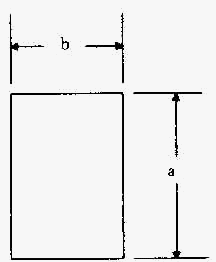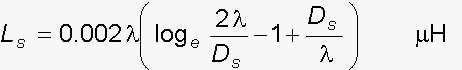where Ls = self inductance, ?H Ds = 0.2235 (a + b) = g.m.d. (geometric mean distance from itself), cm l = length of busbar, cm Two Parallel Conductorswhere M = Mutual inductance, ?H Dm = g.m.d between bars l = length of busbar, cm Geometric Mean Distance Formulae Rectangular Bars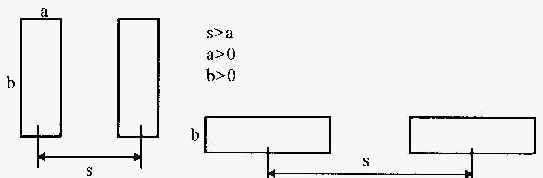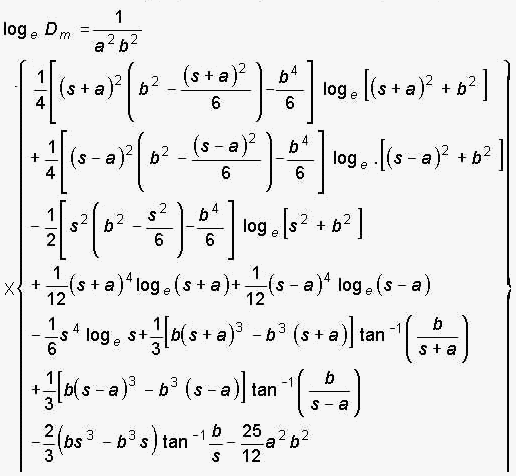For three Phase conductors we can write: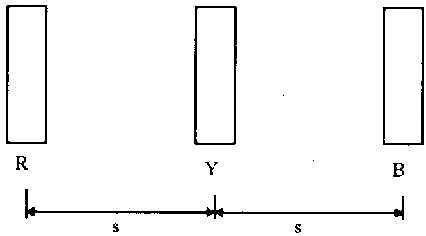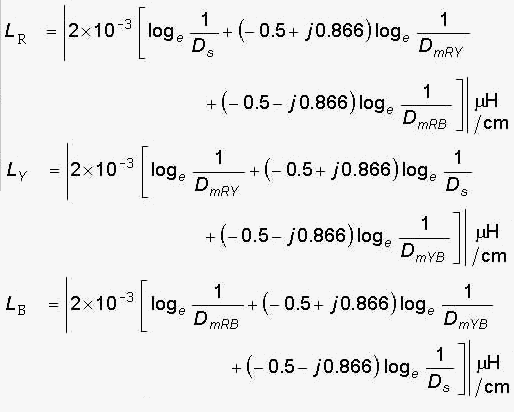Viewing 1 replies (of 1 total)
• You must be logged in to reply to this topic.